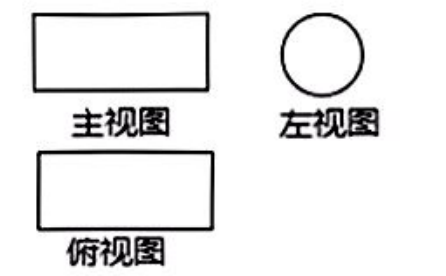$\text{A.}$ 三棱柱 $\text{B.}$ 三棱锥 $\text{C.}$ 圆柱 $\text{D.}$ 圆锥
【答案】 C